Documentation

### This is machine translation

Mouseover text to see original. Click the button below to return to the English version of the page.

To view all translated materials including this page, select Country from the country navigator on the bottom of this page.

# histogram2

Bivariate histogram plot

## Description

Bivariate histograms are a type of bar plot for numeric data that group the data into 2-D bins. After you create a `Histogram2` object, you can modify aspects of the histogram by changing its property values. This is particularly useful for quickly modifying the properties of the bins or changing the display.

## Creation

### Syntax

``histogram2(X,Y)``
``histogram2(X,Y,nbins)``
``histogram2(X,Y,Xedges,Yedges)``
``histogram2('XBinEdges',Xedges,'YBinEdges',Yedges,'BinCounts',counts)``
``histogram2(___,Name,Value)``
``histogram2(ax,___)``
``h = histogram2(___)``

### Description

example

````histogram2(X,Y)` creates a bivariate histogram plot of `X` and `Y`. The `histogram2` function uses an automatic binning algorithm that returns bins with a uniform area, chosen to cover the range of elements in `X` and `Y` and reveal the underlying shape of the distribution. `histogram2` displays the bins as 3-D rectangular bars such that the height of each bar indicates the number of elements in the bin.```

example

````histogram2(X,Y,nbins)` specifies the number of bins to use in each dimension of the histogram.```

example

````histogram2(X,Y,Xedges,Yedges)` specifies the edges of the bins in each dimension using the vectors `Xedges` and `Yedges`.```
````histogram2('XBinEdges',Xedges,'YBinEdges',Yedges,'BinCounts',counts)` manually specifies the bin counts. `histogram2` plots the specified bin counts and does not do any data binning.```

example

````histogram2(___,Name,Value)` specifies additional options with one or more `Name,Value` pair arguments using any of the previous syntaxes. For example, you can specify `'BinWidth'` and a two-element vector to adjust the width of the bins in each dimension, or `'Normalization'` with a valid option (`'count'`, `'probability'`, `'countdensity'`, `'pdf'`, `'cumcount'`, or `'cdf'`) to use a different type of normalization. For a list of properties, see Histogram2 Properties.```
````histogram2(ax,___)` plots into the axes specified by `ax` instead of into the current axes (`gca`). The option `ax` can precede any of the input argument combinations in the previous syntaxes.```

example

````h = histogram2(___)` returns a `Histogram2` object. Use this to inspect and adjust properties of the bivariate histogram. For a list of properties, see Histogram2 Properties.```

### Input Arguments

expand all

Data to distribute among bins, specified as separate arguments of vectors, matrices, or multidimensional arrays. `X` and `Y` must be the same size. If `X` and `Y` are not vectors, then `histogram2` treats them as single column vectors, `X(:)` and `Y(:)`, and plots a single histogram.

Corresponding elements in `X` and `Y` specify the x and y coordinates of 2-D data points, `[X(k),Y(k)]`. The data types of `X` and `Y` can be different, but `histogram2` concatenates these inputs into a single `N`-by-`2` matrix of the dominant data type.

`histogram2` ignores all `NaN` values. Similarly, `histogram2` ignores `Inf` and `-Inf` values, unless the bin edges explicitly specify `Inf` or `-Inf` as a bin edge. Although `NaN`, `Inf`, and `-Inf` values are typically not plotted, they are still included in normalization calculations that include the total number of data elements, such as `'probability'`.

### Note

If `X` or `Y` contain integers of type `int64` or `uint64` that are larger than `flintmax`, then it is recommended that you explicitly specify the histogram bin edges.`histogram2` automatically bins the input data using double precision, which lacks integer precision for numbers greater than `flintmax`.

Data Types: `single` | `double` | `int8` | `int16` | `int32` | `int64` | `uint8` | `uint16` | `uint32` | `uint64` | `logical`

Number of bins in each dimension, specified as a positive scalar integer or two-element vector of positive integers. If you do not specify `nbins`, then `histogram2` automatically calculates how many bins to use based on the values in `X` and `Y`.

• If `nbins` is a scalar, then `histogram2` uses that many bins in each dimension.

• If `nbins` is a vector, then `nbins(1)` specifies the number of bins in the x-dimension and `nbins(2)` specifies the number of bins in the y-dimension.

Example: `histogram2(X,Y,20)` uses 20 bins in each dimension.

Example: `histogram2(X,Y,[10 20])` uses 10 bins in the `x`-dimension and 20 bins in the `y`-dimension.

Bin edges in x-dimension, specified as a vector. `Xedges(1)` is the first edge of the first bin in the x-dimension, and `Xedges(end)` is the outer edge of the last bin.

The value `[X(k),Y(k)]` is in the `(i,j)`th bin if `Xedges(i)``X(k)` < `Xedges(i+1)` and `Yedges(j)``Y(k)` < `Yedges(j+1)`. The last bins in each dimension also include the last (outer) edge. For example, `[X(k),Y(k)]` falls into the `i`th bin in the last row if `Xedges(end-1)``X(k)``Xedges(end)` and `Yedges(i)``Y(k)` < `Yedges(i+1)`.

Data Types: `single` | `double` | `int8` | `int16` | `int32` | `int64` | `uint8` | `uint16` | `uint32` | `uint64` | `logical`

Bin edges in y-dimension, specified as a vector. `Yedges(1)` is the first edge of the first bin in the y-dimension, and `Yedges(end)` is the outer edge of the last bin.

The value `[X(k),Y(k)]` is in the `(i,j)`th bin if `Xedges(i)``X(k)` < `Xedges(i+1)` and `Yedges(j)``Y(k)` < `Yedges(j+1)`. The last bins in each dimension also include the last (outer) edge. For example, `[X(k),Y(k)]` falls into the `i`th bin in the last row if `Xedges(end-1)``X(k)``Xedges(end)` and `Yedges(i)``Y(k)` < `Yedges(i+1)`.

Data Types: `single` | `double` | `int8` | `int16` | `int32` | `int64` | `uint8` | `uint16` | `uint32` | `uint64` | `logical`

Bin counts, specified as a matrix. Use this input to pass bin counts to `histogram2` when the bin counts calculation is performed separately and you do not want `histogram2` to do any data binning.

`counts` must be a matrix of size `[length(XBinEdges)-1 length(YBinEdges)-1]` so that it specifies a bin count for each bin.

Example: ```histogram2('XBinEdges',-1:1,'YBinEdges',-2:2,'BinCounts',[1 2 3 4; 5 6 7 8])```

Axes object. If you do not specify an axes, then the `histogram2` function uses the current axes (`gca`).

#### Name-Value Pair Arguments

Specify optional comma-separated pairs of `Name,Value` arguments. `Name` is the argument name and `Value` is the corresponding value. `Name` must appear inside quotes. You can specify several name and value pair arguments in any order as `Name1,Value1,...,NameN,ValueN`.

Example: `histogram2(X,Y,'BinWidth',[5 10])`

The properties listed here are only a subset. For a complete list, see Histogram2 Properties.

Binning algorithm, specified as one of the values in this table.

ValueDescription
`'auto'`

The default `'auto'` algorithm chooses a bin width to cover the data range and reveal the shape of the underlying distribution.

`'scott'`

Scott’s rule is optimal if the data is close to being jointly normally distributed. This rule is appropriate for most other distributions, as well. It uses a bin size of ```[3.5*std(X(:))*numel(X)^(-1/4), 3.5*std(Y(:))*numel(Y)^(-1/4)]```.

`'fd'`

The Freedman-Diaconis rule is less sensitive to outliers in the data, and might be more suitable for data with heavy-tailed distributions. It uses a bin size of ```[2*IQR(X(:))*numel(X)^(-1/4), 2*IQR(Y(:))*numel(Y)^(-1/4)]```, where `IQR` is the interquartile range.

`'integers'`

The integer rule is useful with integer data, as it creates bins centered on pairs of integers. It uses a bin width of 1 for each dimension and places bin edges halfway between integers.

To avoid accidentally creating too many bins, you can use this rule to create a limit of 1024 bins (210). If the data range for either dimension is greater than 1024, then the integer rule uses wider bins instead.

`histogram2` does not always choose the number of bins using these exact formulas. Sometimes the number of bins is adjusted slightly so that the bin edges fall on "nice" numbers.

### Note

If you set the `NumBins`, `XBinEdges`, `YBinEdges`, `BinWidth`, or `BinLimits` property, then the `BinMethod` property is set to `'manual'`.

Example: `histogram2(X,Y,'BinMethod','integers')` creates a bivariate histogram with the bins centered on pairs of integers.

Width of bins in each dimension, specified as a two-element vector of positive integers, `[xWidth yWidth]`.

If you specify `BinWidth`, then `histogram2` can use a maximum of 1024 bins (210) along each dimension. If instead the specified bin width requires more bins, then `histogram2` uses a larger bin width corresponding to the maximum number of bins.

Example: `histogram2(X,Y,'BinWidth',[5 10])` uses bins with size `5` in the `x`-dimension and size `10` in the `y`-dimension.

Histogram display style, specified as either `'bar3'` or `'tile'`. Specify `'tile'` to display the histogram as a rectangular array of tiles with colors indicating the bin values.

The default value of `'bar3'` displays the histogram using 3-D bars.

Example: `histogram2(X,Y,'DisplayStyle','tile')` plots the histogram as a rectangular array of tiles.

Transparency of histogram bar edges, specified as a scalar value between `0` and `1` inclusive. A value of `1` means fully opaque and `0` means completely transparent (invisible).

Example: `histogram2(X,Y,'EdgeAlpha',0.5)` creates a bivariate histogram plot with semi-transparent bar edges.

Histogram edge color, specified as one of these values:

• `'none'` — Edges are not drawn.

• `'auto'` — Color of each edge is chosen automatically.

• RGB triplet, hexadecimal color code, or color name — Edges use the specified color.

RGB triplets and hexadecimal color codes are useful for specifying custom colors.

• An RGB triplet is a three-element row vector whose elements specify the intensities of the red, green, and blue components of the color. The intensities must be in the range `[0,1]`; for example,``` [0.4 0.6 0.7]```.

• A hexadecimal color code is a character vector or a string scalar that starts with a hash symbol (`#`) followed by three or six hexadecimal digits, which can range from `0` to `F`. The values are not case sensitive. Thus, the color codes `'#FF8800'`, `'#ff8800'`, `'#F80'`, and `'#f80'` are equivalent.

Alternatively, you can specify some common colors by name. This table lists the named color options, the equivalent RGB triplets, and hexadecimal color codes.

Color NameShort NameRGB TripletHexadecimal Color CodeAppearance
`'red'``'r'``[1 0 0]``'#FF0000'``'green'``'g'``[0 1 0]``'#00FF00'``'blue'``'b'``[0 0 1]``'#0000FF'``'cyan'` `'c'``[0 1 1]``'#00FFFF'``'magenta'``'m'``[1 0 1]``'#FF00FF'``'yellow'``'y'``[1 1 0]``'#FFFF00'``'black'``'k'``[0 0 0]``'#000000'``'white'``'w'``[1 1 1]``'#FFFFFF'`Here are the RGB triplets and hexadecimal color codes for the default colors MATLAB® uses in many types of plots.

RGB TripletHexadecimal Color CodeAppearance
`[0 0.4470 0.7410]``'#0072BD'``[0.8500 0.3250 0.0980]``'#D95319'``[0.9290 0.6940 0.1250]``'#EDB120'``[0.4940 0.1840 0.5560]``'#7E2F8E'``[0.4660 0.6740 0.1880]``'#77AC30'``[0.3010 0.7450 0.9330]``'#4DBEEE'``[0.6350 0.0780 0.1840]``'#A2142F'`Example: `histogram2(X,Y,'EdgeColor','r')` creates a 3-D histogram plot with red bar edges.

Transparency of histogram bars, specified as a scalar value between `0` and `1` inclusive. `histogram2` uses the same transparency for all the bars of the histogram. A value of `1` means fully opaque and `0` means completely transparent (invisible).

Example: `histogram2(X,Y,'FaceAlpha',0.5)` creates a bivariate histogram plot with semi-transparent bars.

Histogram bar color, specified as one of these values:

• `'none'` — Bars are not filled.

• `'flat'` — Bar colors vary with height. Bars with different height have different colors. The colors are selected from the figure or axes colormap.

• `'auto'` — Bar color is chosen automatically (default).

• RGB triplet, hexadecimal color code, or color name — Bars are filled with the specified color.

RGB triplets and hexadecimal color codes are useful for specifying custom colors.

• An RGB triplet is a three-element row vector whose elements specify the intensities of the red, green, and blue components of the color. The intensities must be in the range `[0,1]`; for example,``` [0.4 0.6 0.7]```.

• A hexadecimal color code is a character vector or a string scalar that starts with a hash symbol (`#`) followed by three or six hexadecimal digits, which can range from `0` to `F`. The values are not case sensitive. Thus, the color codes `'#FF8800'`, `'#ff8800'`, `'#F80'`, and `'#f80'` are equivalent.

Alternatively, you can specify some common colors by name. This table lists the named color options, the equivalent RGB triplets, and hexadecimal color codes.

Color NameShort NameRGB TripletHexadecimal Color CodeAppearance
`'red'``'r'``[1 0 0]``'#FF0000'``'green'``'g'``[0 1 0]``'#00FF00'``'blue'``'b'``[0 0 1]``'#0000FF'``'cyan'` `'c'``[0 1 1]``'#00FFFF'``'magenta'``'m'``[1 0 1]``'#FF00FF'``'yellow'``'y'``[1 1 0]``'#FFFF00'``'black'``'k'``[0 0 0]``'#000000'``'white'``'w'``[1 1 1]``'#FFFFFF'`Here are the RGB triplets and hexadecimal color codes for the default colors MATLAB uses in many types of plots.

RGB TripletHexadecimal Color CodeAppearance
`[0 0.4470 0.7410]``'#0072BD'``[0.8500 0.3250 0.0980]``'#D95319'``[0.9290 0.6940 0.1250]``'#EDB120'``[0.4940 0.1840 0.5560]``'#7E2F8E'``[0.4660 0.6740 0.1880]``'#77AC30'``[0.3010 0.7450 0.9330]``'#4DBEEE'``[0.6350 0.0780 0.1840]``'#A2142F'`If you specify `DisplayStyle` as `'stairs'`, then `histogram2` does not use the `FaceColor` property.

Example: `histogram2(X,Y,'FaceColor','g')` creates a 3-D histogram plot with green bars.

Lighting effect on histogram bars, specified as one of the values in this table.

ValueDescription
`'lit'`

Histogram bars display a pseudo-lighting effect, where the sides of the bars use darker colors relative to the tops. The bars are unaffected by other light sources in the axes.

This is the default value when `DisplayStyle` is `'bar3'`.

`'flat'`

Histogram bars are not lit automatically. In the presence of other light objects, the lighting effect is uniform across the bar faces.

`'none'`

Histogram bars are not lit automatically, and lights do not affect the histogram bars.

`FaceLighting` can only be `'none'` when `DisplayStyle` is `'tile'`.

Example: `histogram2(X,Y,'FaceLighting','none')` turns off the lighting of the histogram bars.

Line style, specified as one of the options listed in this table.

Line StyleDescriptionResulting Line
`'-'`Solid line`'--'`Dashed line`':'`Dotted line`'-.'`Dash-dotted line`'none'`No lineNo line

Width of bar outlines, specified as a positive value in point units. One point equals 1/72 inch.

Example: `1.5`

Data Types: `single` | `double` | `int8` | `int16` | `int32` | `int64` | `uint8` | `uint16` | `uint32` | `uint64`

Type of normalization, specified as one of the values in this table. For each bin `i`:

• ${v}_{i}$ is the bin value.

• ${c}_{i}$ is the number of elements in the bin.

• ${A}_{i}={w}_{xi}\cdot {w}_{yi}$ is the area of each bin, computed using the x and y bin widths.

• $N$ is the number of elements in the input data. This value can be greater than the binned data if the data contains `NaN` values, or if some of the data lies outside the bin limits.

ValueBin ValuesNotes
`'count'` (default)

`${v}_{i}={c}_{i}$`

• Count or frequency of observations.

• Sum of bin values is less than or equal to `numel(X)` and `numel(y)`. The sum is less than `numel(X)` and `numel(y)` only when some of the input data is not included in the bins.

`'countdensity'`

`${v}_{i}=\frac{{c}_{i}}{{A}_{i}}$`

• Count or frequency scaled by area of bin.

• The volume (height * area) of each bar is the number of observations in the bin. The sum of the bar volumes is less than or equal to `numel(X)` and `numel(y)`.

`'cumcount'`

`${v}_{i}=\sum _{j=1}^{i}{c}_{j}$`

• Cumulative count. Each bin value is the cumulative number of observations in each bin and all previous bins in both the x and y dimensions.

• The height of the last bar is less than or equal to `numel(X)` and `numel(Y)`.

`'probability'`

`${v}_{i}=\frac{{c}_{i}}{N}$`

• Relative probability.

• The sum of the bar heights is less than or equal to `1`.

`'pdf'`

`${v}_{i}=\frac{{c}_{i}}{N\cdot {A}_{i}}$`

• Probability density function estimate.

• The volume of each bar is the relative number of observations. The sum of the bar volumes is less than or equal to `1`.

`'cdf'`

`${v}_{i}=\sum _{j=1}^{i}\text{\hspace{0.17em}}\frac{{c}_{j}}{N}$`

• Cumulative density function estimate.

• The height of each bar is equal to the cumulative relative number of observations in each bin and all previous bins in both the x and y dimensions. The height of the last bar is less than or equal to `1`.

Example: `histogram2(X,Y,'Normalization','pdf')` plots an estimate of the probability density function for `X` and `Y`.

Toggle display of empty bins, specified as either `'off'` or `'on'`. The default value is `'off'`.

Example: `histogram2(X,Y,'ShowEmptyBins','on')` turns on the display of empty bins.

Bin limits in x-dimension, specified as a two-element vector, `[xbmin,xbmax]`. The vector indicates the first and last bin edges in the x-dimension.

`histogram2` only plots data that falls within the bin limits inclusively, ```Data(Data(:,1)>=xbmin & Data(:,1)<=xbmax)```.

Selection mode for bin limits in x-dimension, specified as `'auto'` or `'manual'`. The default value is `'auto'`, so that the bin limits automatically adjust to the data along the x-axis.

If you explicitly specify either `XBinLimits` or `XBinEdges`, then `XBinLimitsMode` is set automatically to `'manual'`. In that case, specify `XBinLimitsMode` as `'auto'` to rescale the bin limits to the data.

Bin limits in y-dimension, specified as a two-element vector, `[ybmin,ybmax]`. The vector indicates the first and last bin edges in the y-dimension.

`histogram2` only plots data that falls within the bin limits inclusively, ```Data(Data(:,2)>=ybmin & Data(:,2)<=ybmax)```.

Selection mode for bin limits in y-dimension, specified as `'auto'` or `'manual'`. The default value is `'auto'`, so that the bin limits automatically adjust to the data along the y-axis.

If you explicitly specify either `YBinLimits` or `YBinEdges`, then `YBinLimitsMode` is set automatically to `'manual'`. In that case, specify `YBinLimitsMode` as `'auto'` to rescale the bin limits to the data.

### Output Arguments

expand all

Bivariate histogram, returned as an object. For more information, see Histogram2 Properties.

## Properties

 Histogram2 Properties Histogram2 appearance and behavior

## Object Functions

 `morebins` Increase number of histogram bins `fewerbins` Decrease number of histogram bins

## Examples

collapse all

Generate 10,000 pairs of random numbers and create a bivariate histogram. The `histogram2` function automatically chooses an appropriate number of bins to cover the range of values in `x` and `y` and show the shape of the underlying distribution.

```x = randn(10000,1); y = randn(10000,1); h = histogram2(x,y)```
```h = Histogram2 with properties: Data: [10000x2 double] Values: [25x28 double] NumBins: [25 28] XBinEdges: [1x26 double] YBinEdges: [1x29 double] BinWidth: [0.3000 0.3000] Normalization: 'count' FaceColor: 'auto' EdgeColor: [0.1500 0.1500 0.1500] Show all properties ```
```xlabel('x') ylabel('y')```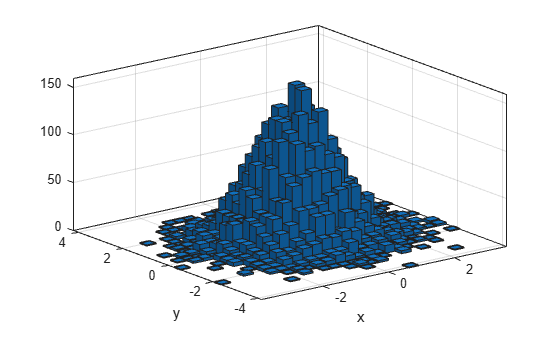When you specify an output argument to the `histogram2` function, it returns a histogram2 object. You can use this object to inspect the properties of the histogram, such as the number of bins or the width of the bins.

Find the number of histogram bins in each dimension.

`nXnY = h.NumBins`
```nXnY = 1×2 25 28 ```

Plot a bivariate histogram of 1,000 pairs of random numbers sorted into 25 equally spaced bins, using 5 bins in each dimension.

```x = randn(1000,1); y = randn(1000,1); nbins = 5; h = histogram2(x,y,nbins)``````h = Histogram2 with properties: Data: [1000x2 double] Values: [5x5 double] NumBins: [5 5] XBinEdges: [-4 -2.4000 -0.8000 0.8000 2.4000 4] YBinEdges: [-4 -2.4000 -0.8000 0.8000 2.4000 4] BinWidth: [1.6000 1.6000] Normalization: 'count' FaceColor: 'auto' EdgeColor: [0.1500 0.1500 0.1500] Show all properties ```

Find the resulting bin counts.

`counts = h.Values`
```counts = 5×5 0 2 3 1 0 2 40 124 47 4 1 119 341 109 10 1 32 117 33 1 0 4 8 1 0 ```

Generate 1,000 pairs of random numbers and create a bivariate histogram.

```x = randn(1000,1); y = randn(1000,1); h = histogram2(x,y)``````h = Histogram2 with properties: Data: [1000x2 double] Values: [15x15 double] NumBins: [15 15] XBinEdges: [1x16 double] YBinEdges: [1x16 double] BinWidth: [0.5000 0.5000] Normalization: 'count' FaceColor: 'auto' EdgeColor: [0.1500 0.1500 0.1500] Show all properties ```

Use the `morebins` function to coarsely adjust the number of bins in the x dimension.

```nbins = morebins(h,'x'); nbins = morebins(h,'x')``````nbins = 1×2 19 15 ```

Use the `fewerbins` function to adjust the number of bins in the y dimension.

```nbins = fewerbins(h,'y'); nbins = fewerbins(h,'y')``````nbins = 1×2 19 11 ```

Adjust the number of bins at a fine grain level by explicitly setting the number of bins.

`h.NumBins = [20 10];`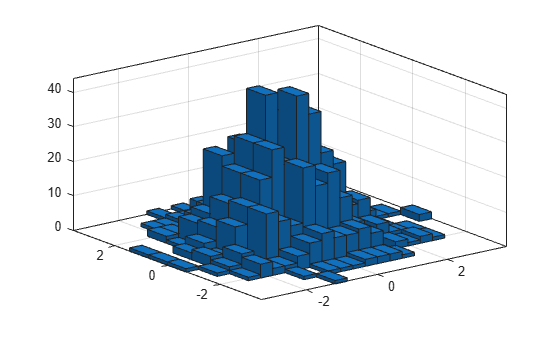Create a bivariate histogram using 1,000 normally distributed random numbers with 12 bins in each dimension. Specify `FaceColor` as `'flat'` to color the histogram bars by height.

```h = histogram2(randn(1000,1),randn(1000,1),[12 12],'FaceColor','flat'); colorbar```Generate random data and plot a bivariate tiled histogram. Display the empty bins by specifying `ShowEmptyBins` as `'on'`.

```x = 2*randn(1000,1)+2; y = 5*randn(1000,1)+3; h = histogram2(x,y,'DisplayStyle','tile','ShowEmptyBins','on');```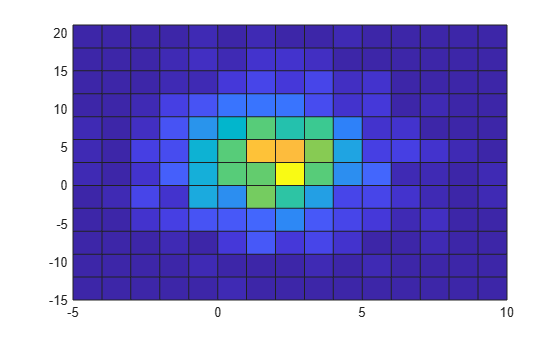Generate 1,000 pairs of random numbers and create a bivariate histogram. Specify the bin edges using two vectors, with infinitely wide bins on the boundary of the histogram to capture all outliers that do not satisfy $|x|<2$.

```x = randn(1000,1); y = randn(1000,1); Xedges = [-Inf -2:0.4:2 Inf]; Yedges = [-Inf -2:0.4:2 Inf]; h = histogram2(x,y,Xedges,Yedges)``````h = Histogram2 with properties: Data: [1000x2 double] Values: [12x12 double] NumBins: [12 12] XBinEdges: [1x13 double] YBinEdges: [1x13 double] BinWidth: 'nonuniform' Normalization: 'count' FaceColor: 'auto' EdgeColor: [0.1500 0.1500 0.1500] Show all properties ```

When the bin edges are infinite, `histogram2` displays each outlier bin (along the boundary of the histogram) as being double the width of the bin next to it.

Specify the `Normalization` property as `'countdensity'` to remove the bins containing the outliers. Now the volume of each bin represents the frequency of observations in that interval.

`h.Normalization = 'countdensity';`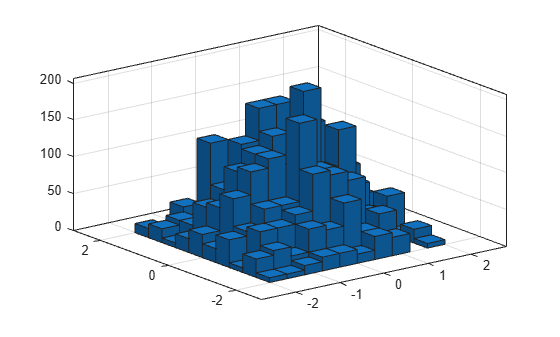Generate 1,000 pairs of random numbers and create a bivariate histogram using the `'probability'` normalization.

```x = randn(1000,1); y = randn(1000,1); h = histogram2(x,y,'Normalization','probability')``````h = Histogram2 with properties: Data: [1000x2 double] Values: [15x15 double] NumBins: [15 15] XBinEdges: [1x16 double] YBinEdges: [1x16 double] BinWidth: [0.5000 0.5000] Normalization: 'probability' FaceColor: 'auto' EdgeColor: [0.1500 0.1500 0.1500] Show all properties ```

Compute the total sum of the bar heights. With this normalization, the height of each bar is equal to the probability of selecting an observation within that bin interval, and the heights of all of the bars sum to 1.

`S = sum(h.Values(:))`
```S = 1.0000 ```

Generate 1,000 pairs of random numbers and create a bivariate histogram. Return the histogram object to adjust the properties of the histogram without recreating the entire plot.

```x = randn(1000,1); y = randn(1000,1); h = histogram2(x,y)``````h = Histogram2 with properties: Data: [1000x2 double] Values: [15x15 double] NumBins: [15 15] XBinEdges: [1x16 double] YBinEdges: [1x16 double] BinWidth: [0.5000 0.5000] Normalization: 'count' FaceColor: 'auto' EdgeColor: [0.1500 0.1500 0.1500] Show all properties ```

Color the histogram bars by height.

`h.FaceColor = 'flat';`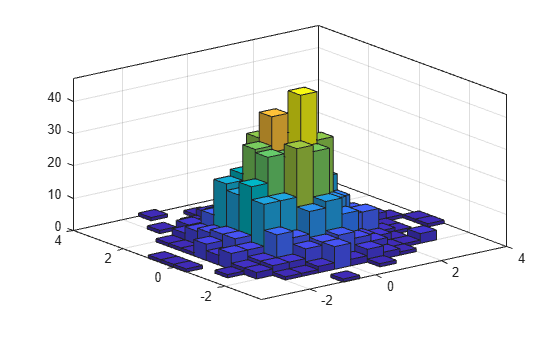Change the number of bins in each direction.

`h.NumBins = [10 25];`Display the histogram as a tile plot.

```h.DisplayStyle = 'tile'; view(2)```Use the `savefig` function to save a histogram2 figure.

```y = histogram2(randn(100,1),randn(100,1)); savefig('histogram2.fig');```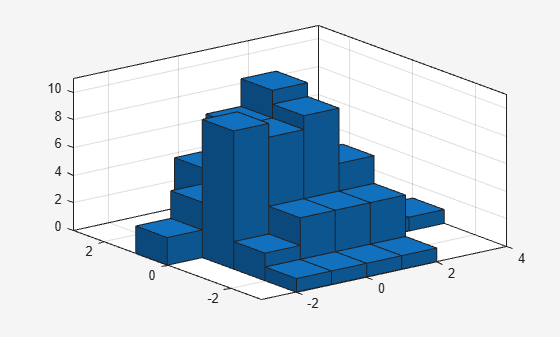```clear all close all```

Use `openfig` to load the histogram figure back into MATLAB. `openfig` also returns a handle to the figure, `h`.

`h = openfig('histogram2.fig');`

Use the `findobj` function to locate the correct object handle from the figure handle. This allows you to continue manipulating the original histogram object used to generate the figure.

`y = findobj(h, 'type', 'histogram2')`
```y = Histogram2 with properties: Data: [100x2 double] Values: [7x6 double] NumBins: [7 6] XBinEdges: [-3 -2 -1 0 1 2 3 4] YBinEdges: [-3 -2 -1 0 1 2 3] BinWidth: [1 1] Normalization: 'count' FaceColor: 'auto' EdgeColor: [0.1500 0.1500 0.1500] Show all properties ```

## Tips

• Histogram plots created using `histogram2` have a context menu in plot edit mode that enables interactive manipulations in the figure window. For example, you can use the context menu to interactively change the number of bins, align multiple histograms, or change the display order.